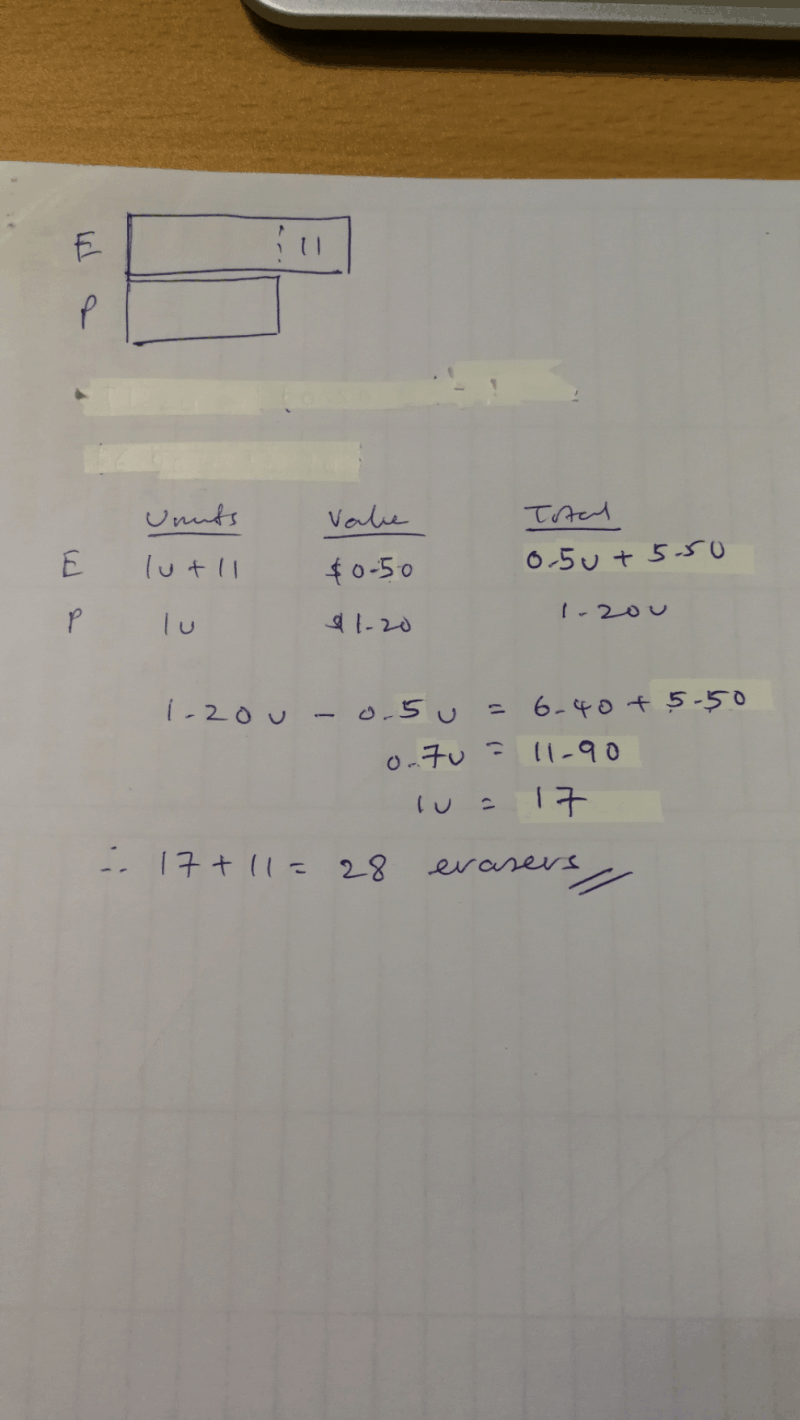# Question

A bookshop sold pens at \$1.20 each and erasers at \$0.50 each.  A teacher bought some pens and erasers for her pupils.  She spent \$6.40 more on pens but she has 11 pens fewer than erasers.  How many erasers did the teacher buy?

Hi Xueyan

Let me try to help you out with this question by giving you some explanation and also without fancy models or diagrams.

So we understand that there are 11 more erasers than pens, since there are 11 fewer pens than erasers? We know that the total cost of these 11 erasers is going to be \$5.50, because 11 x \$0.50 is \$5.50.

Now if we remove these 11 erasers and set them aside, and we look at the number and also the costs of the items again, we will notice that there are now the same number of pens and erasers. However, because we have now set aside the other 11 erasers just now, and ignoring them for now, we know that all the pens costs \$6.40 + \$5.50 = \$11.90 more than the remaining erasers. Now let’s also leave this aside for now.

So now, we have an equal number of pens and erasers right? What we are going to do, is we will be able to pair up one eraser and one pen. (yes we don’t know the exact number of pens and erasers yet, we will get to that soon.) With a pair of eraser and a pen, we notice that the difference in value is \$0.70. What we can see is that, in one pair of eraser and pen, the pen costs \$0.70 more than the eraser.

So if we have 2 pairs of erasers and pens, the difference will be \$1.40.

If we have 3 pairs of erasers and pens, the difference will be \$2.10.

But remember, we said that the pens actually costs \$11.90 more than the remaining erasers?

So that means, if we take \$11.90 divided by \$0.70, we will be able to find out that there are 17 pairs of erasers and pens!

So now we know that there are 17 erasers in pairs, we will take into account the 11 erasers that we have left out just now, and we will add them all up.

We now know that there are 17 + 11 = 28 erasers.

Hope this gives you a better way of viewing the question and solution and it clarifies things.

We provide coaching for small, focus groups of students at Future Achievers Hub at Tampines Junction.

Do check out:

www.futureachievers.com.sg

or call us at +65 83325521,